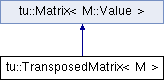CMR  1.3.0
tu::TransposedMatrix< M > Class Template Reference

Matrix proxy for the transpose of a matrix. More...

#include <matrix.hpp>

Inheritance diagram for tu::TransposedMatrix< M >:## Classes

class  NonzeroIterator

## Public Types

typedef M::Value Value

typedef M::Index Index

typedef M::Nonzero Nonzero

typedef detail::Range< NonzeroIterator< true > > NonzeroRowRange

typedef detail::Range< NonzeroIterator< false > > NonzeroColumnRangePublic Types inherited from tu::Matrix< M::Value >
typedef M::Value Value

typedef std::size_t Index
Type of entries. More...

## Public Member Functions

TransposedMatrix (M &matrix)
Constructs from a matrix of type M. More...

TransposedMatrix (TransposedMatrix &&other)
Move constructor. More...

std::size_t numRows () const
Returns the number of rows. More...

std::size_t numColumns () const
Returns the number of columns. More...

bool hasSortedRows () const
Indicates if the row data is sorted by column. More...

bool hasSortedColumns () const
Indicates if the column data is sorted by row. More...

const Valueget (Index row, Index column) const
Returns entry at row, column. More...

std::size_t numNonzeros () const
Returns the number of nonzeros. More...

std::size_t countRowNonzeros (Index row) const
Returns the number of nonzeros in row. More...

std::size_t countColumnNonzeros (Index column) const
Returns the number of nonzeros in column. More...

NonzeroRowRange iterateRowNonzeros (Index row) const
Returns a range for iterating over the nonzeros of row. More...

NonzeroColumnRange iterateColumnNonzeros (Index column) const
Returns a range for iterating over the nonzeros of column. More...

void ensureConsistency () const
Checks for consistency, raising a std::runtime_error if inconsistent. More...

M & _matrix

## Detailed Description

### template<typename M> class tu::TransposedMatrix< M >

Matrix proxy for the transpose of a matrix.

Matrix proxy for the transpose of a matrix. It references another matrix and does not maintain matrix data by itself.

## Member Typedef Documentation

template<typename M>
 typedef M::Index tu::TransposedMatrix< M >::Index
template<typename M>
 typedef M::Nonzero tu::TransposedMatrix< M >::Nonzero
template<typename M>
 typedef detail::Range > tu::TransposedMatrix< M >::NonzeroColumnRange
template<typename M>
 typedef detail::Range > tu::TransposedMatrix< M >::NonzeroRowRange
template<typename M>
 typedef M::Value tu::TransposedMatrix< M >::Value

## Constructor & Destructor Documentation

template<typename M>
 tu::TransposedMatrix< M >::TransposedMatrix ( M & matrix )
inline

Constructs from a matrix of type M.

template<typename M>
 tu::TransposedMatrix< M >::TransposedMatrix ( TransposedMatrix< M > && other )
inline

Move constructor.

## Member Function Documentation

template<typename M>
 std::size_t tu::TransposedMatrix< M >::countColumnNonzeros ( Index column ) const
inline

Returns the number of nonzeros in column.

template<typename M>
 std::size_t tu::TransposedMatrix< M >::countRowNonzeros ( Index row ) const
inline

Returns the number of nonzeros in row.

template<typename M>
 void tu::TransposedMatrix< M >::ensureConsistency ( ) const
inline

Checks for consistency, raising a std::runtime_error if inconsistent.

template<typename M>
 const Value& tu::TransposedMatrix< M >::get ( Index row, Index column ) const
inline

Returns entry at row, column.

Returns entry at row, column. If the row or column data is sorted (resp. not sorted) then the time is logarithmic (resp. linear) in the number of nonzeros of the row or column.

template<typename M>
 bool tu::TransposedMatrix< M >::hasSortedColumns ( ) const
inline

Indicates if the column data is sorted by row.

template<typename M>
 bool tu::TransposedMatrix< M >::hasSortedRows ( ) const
inline

Indicates if the row data is sorted by column.

template<typename M>
 NonzeroColumnRange tu::TransposedMatrix< M >::iterateColumnNonzeros ( Index column ) const
inline

Returns a range for iterating over the nonzeros of column.

template<typename M>
 NonzeroRowRange tu::TransposedMatrix< M >::iterateRowNonzeros ( Index row ) const
inline

Returns a range for iterating over the nonzeros of row.

template<typename M>
 std::size_t tu::TransposedMatrix< M >::numColumns ( ) const
inline

Returns the number of columns.

template<typename M>
 std::size_t tu::TransposedMatrix< M >::numNonzeros ( ) const
inline

Returns the number of nonzeros.

template<typename M>
 std::size_t tu::TransposedMatrix< M >::numRows ( ) const
inline

Returns the number of rows.

## Member Data Documentation

template<typename M>
 M& tu::TransposedMatrix< M >::_matrix
protected

The documentation for this class was generated from the following file:
• /home/travis/build/discopt/cmr/src/cmr/matrix.hpp